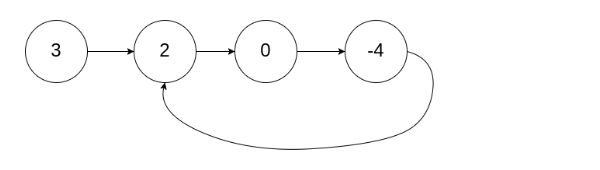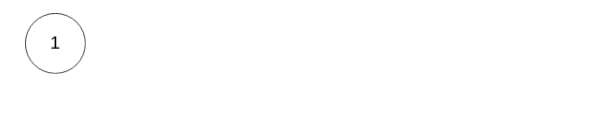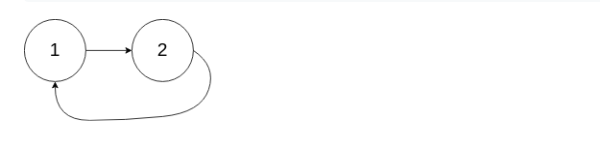15

Cycle Detection in a Singly Linked List

Difficulty: MEDIUM
Avg. time to solve
15 min
Success Rate
80%

Problem Statement

Note: Since, it is binary problem, there is no partial marking. Marks will only be awarded if you get all the test cases correct.

Input format :
The first line of each test case contains the elements of the singly linked list separated by a single space and terminated by -1 and hence -1 would never be a list element.

The second line contains the integer position "pos" which represents the position (0-indexed) in the linked list where tail connects to. If "pos" is -1, then there is no cycle in the linked list.
Output format :
The only line of output contains 'true' if linked list has a cycle or 'false' otherwise.

You don't have to explicitly print by yourself. It has been taken care of.
Constraints :
0 <= N <= 10^6
-1 <= pos < N
-10^9 <= data <= 10^9 and data != -1

Where 'N' is the size of the singly linked list, "pos" represents the position (0-indexed) in the linked list where tail connects to and "data" is the Integer data of singly linked list.

Time Limit: 1 sec
Note :
Try to solve this problem in O(N) Time Complexity and O(1) space Complexity
Sample Input 1 :
3 2 0 -4 -1
1true
Sample Input 2 :
1 -1
-1false
Sample Input 3 :
1 2 -1
1Sample Output 3 :
trueConsole